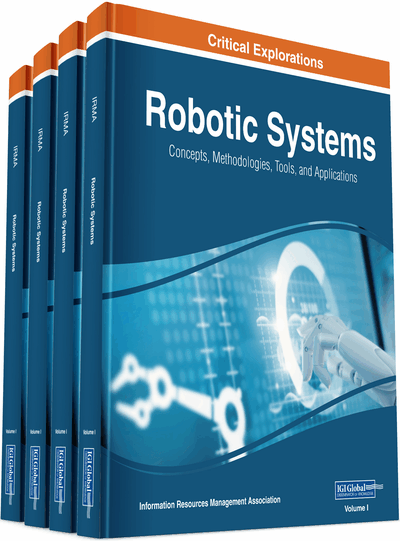A Parallel Levenberg-Marquardt Algorithm for Recursive Neural Network in a Robot Control System

Wei Wang (Jimei University, China), Yunming Pu (Jimei University, China) and Wang Li (Jimei University, China)
DOI: 10.4018/978-1-7998-1754-3.ch038
Available
\$37.50
No Current Special Offers

Abstract

This article has the purpose of overcoming the shortcomings of the recursive neural network learning algorithm and the inherent delay problem on the manipulator master control system. This is by analyzing the shortcomings of LM learning algorithms based on DRNN network, an improved parallel LM algorithm is proposed. The parallel search of the damping coefficient β is found in order to reduce the number of iterations of the loop, and the algorithm is used to decompose the parameter operation and the matrix operation into the processor (core), thereby improve the learning convergence speed, and control the scale of the delay. The simulation results show that the pro-posed algorithm is feasible.
Chapter Preview
Top

The main task of manipulator control is to solve the robot in the work space in the movement of the location, trajectory and attitude, operation sequence and time control and other issues. The most important and most basic is the Invesrekinematics and Invesredynamcis problems and the corresponding control strategies (Oscar, 2002). If we achieve effective control, we need to solve two problems, one is how to achieve the stability of the closed-loop error system, making the trajectory tracking error as soon as possible close to 0; the other is how to suppress interference, as far as possible to reduce the interference signal on the tracking accuracy. For the above problems, the general method is modeled by mathematical methods, and through the linear servo control theory to design the controller, you can get a solution. However, due to the particularity of the robot (uncertainty factors and excessive interference signal), it is difficult to obtain accurate mathematical model, there is no precise mathematical model, it cannot guarantee the quality of control. Until recently, with the increasingly sophisticated neural network control technology, the control problem of the robot seems to have a new opportunity.

Complete Chapter List

Search this Book:
Reset